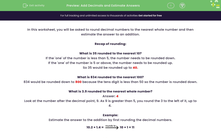# Round Decimals to Nearest Whole Number and Estimate Answers

In this worksheet, students will be asked to round decimal numbers to the nearest whole number and then estimate the answer to an addition.Key stage:  KS 2

Curriculum topic:   Number: Addition, Subtraction, Multiplication and Division

Curriculum subtopic:   Use Estimation to Check Answers

Popular topics:   Decimals worksheets, Estimation worksheets

Difficulty level:#### Worksheet Overview

In this activity, we will be rounding decimal numbers to the nearest whole number and then estimating the answer to an addition.

Recap of rounding

What is 35 rounded to the nearest 10?

If the number in the ones column is less than 5, the number needs to be rounded down.

If the number in the ones column is 5 or above, the number needs to be rounded up.

So 35 would be rounded up to 40.

What is 834 rounded to the nearest 100?

If the number in the tens column is less than 5, the number needs to be rounded down.

If the number in the tens column is 5 or above, the  number needs to be rounded up.

834 would be rounded down to 800 because the tens digit is less than 5.

What is 3.9 rounded to the nearest whole number?

If the number in the tenths column (the first one after the decimal point) is less than 5, the number rounds down.

If the number in the tenths column is 5 or above, the number rounds up.

3.9 has a 9 in the tenths column, so it rounds up to 4.Example

Estimate the answer to the addition by first rounding the decimal numbers to the nearest whole number.

10.2 + 1.4

10.2 has a 2 in the tenths column, and 1.4 has a 4 in the tenths column, so they both round down

10.2 + 1.4 =10 + 1 = 11### What is EdPlace?

We're your National Curriculum aligned online education content provider helping each child succeed in English, maths and science from year 1 to GCSE. With an EdPlace account you’ll be able to track and measure progress, helping each child achieve their best. We build confidence and attainment by personalising each child’s learning at a level that suits them.

Get started#### Popular Maths topics

••••